## Code Correction...

Please I am having problem with this code particularly the last subroutine

#subroutine 1

restart;
Digits:=30:

f:=proc(n)
-25*y[n]+12*cos(x[n]):
end proc:

#subroutine 2

e1:=y[n+4] = -y[n]+2*y[n+2]+((1/15)*h^2+(2/945)*h^2*u^2+(1/56700)*h^2*u^4-(1/415800)*h^2*u^6-(167/833976000)*h^2*u^8-(2633/245188944000)*h^2*u^10-(2671/5557616064000)*h^2*u^12-(257857/13304932857216000)*h^2*u^14-(3073333/4215002729166028800)*h^2*u^16)*f(n)+((16/15)*h^2-(8/945)*h^2*u^2-(1/14175)*h^2*u^4+(1/103950)*h^2*u^6+(167/208494000)*h^2*u^8+(2633/61297236000)*h^2*u^10+(2671/1389404016000)*h^2*u^12+(257857/3326233214304000)*h^2*u^14+(3073333/1053750682291507200)*h^2*u^16)*f(n+1)+((26/15)*h^2+(4/315)*h^2*u^2+(1/9450)*h^2*u^4-(1/69300)*h^2*u^6-(167/138996000)*h^2*u^8-(2633/40864824000)*h^2*u^10-(2671/926269344000)*h^2*u^12-(257857/2217488809536000)*h^2*u^14-(3073333/702500454861004800)*h^2*u^16)*f(n+2)+((16/15)*h^2-(8/945)*h^2*u^2-(1/14175)*h^2*u^4+(1/103950)*h^2*u^6+(167/208494000)*h^2*u^8+(2633/61297236000)*h^2*u^10+(2671/1389404016000)*h^2*u^12+(257857/3326233214304000)*h^2*u^14+(3073333/1053750682291507200)*h^2*u^16)*f(n+3)+((1/15)*h^2+(2/945)*h^2*u^2+(1/56700)*h^2*u^4-(1/415800)*h^2*u^6-(167/833976000)*h^2*u^8-(2633/245188944000)*h^2*u^10-(2671/5557616064000)*h^2*u^12-(257857/13304932857216000)*h^2*u^14-(3073333/4215002729166028800)*h^2*u^16)*f(n+4):

e2:=y[n+3] = -y[n+1]+2*y[n+2]+(-(1/240)*h^2-(31/60480)*h^2*u^2-(67/1814400)*h^2*u^4-(109/53222400)*h^2*u^6-(18127/186810624000)*h^2*u^8-(64931/15692092416000)*h^2*u^10-(9701/59281238016000)*h^2*u^12-(20832397/3406062811447296000)*h^2*u^14-(11349439/51876956666658816000)*h^2*u^16)*f(n)+((1/10)*h^2+(31/15120)*h^2*u^2+(67/453600)*h^2*u^4+(109/13305600)*h^2*u^6+(18127/46702656000)*h^2*u^8+(64931/3923023104000)*h^2*u^10+(9701/14820309504000)*h^2*u^12+(20832397/851515702861824000)*h^2*u^14+(11349439/12969239166664704000)*h^2*u^16)*f(n+1)+((97/120)*h^2-(31/10080)*h^2*u^2-(67/302400)*h^2*u^4-(109/8870400)*h^2*u^6-(18127/31135104000)*h^2*u^8-(64931/2615348736000)*h^2*u^10-(9701/9880206336000)*h^2*u^12-(20832397/567677135241216000)*h^2*u^14-(11349439/8646159444443136000)*h^2*u^16)*f(n+2)+((1/10)*h^2+(31/15120)*h^2*u^2+(67/453600)*h^2*u^4+(109/13305600)*h^2*u^6+(18127/46702656000)*h^2*u^8+(64931/3923023104000)*h^2*u^10+(9701/14820309504000)*h^2*u^12+(20832397/851515702861824000)*h^2*u^14+(11349439/12969239166664704000)*h^2*u^16)*f(n+3)+(-(1/240)*h^2-(31/60480)*h^2*u^2-(67/1814400)*h^2*u^4-(109/53222400)*h^2*u^6-(18127/186810624000)*h^2*u^8-(64931/15692092416000)*h^2*u^10-(9701/59281238016000)*h^2*u^12-(20832397/3406062811447296000)*h^2*u^14-(11349439/51876956666658816000)*h^2*u^16)*f(n+4):

e3:=h*delta[n] = (-149/42-(16/245)*u^2-(1324/169785)*u^4-(559246/695269575)*u^6-(14310311/175207932900)*u^8-(170868550903/20641246574949000)*u^10)*y[n]+(128/21+(32/245)*u^2+(2648/169785)*u^4+(1118492/695269575)*u^6+(14310311/87603966450)*u^8+(170868550903/10320623287474500)*u^10)*y[n+1]+(-107/42-(16/245)*u^2-(1324/169785)*u^4-(559246/695269575)*u^6-(14310311/175207932900)*u^8-(170868550903/20641246574949000)*u^10)*y[n+2]+(-(67/1260)*h^2+(1241/198450)*h^2*u^2+(277961/366735600)*h^2*u^4+(26460409/333729396000)*h^2*u^6+(1363374533/168199615584000)*h^2*u^8+(16323847966961/19815596711951040000)*h^2*u^10)*f(n)+((188/105)*h^2+(5078/99225)*h^2*u^2+(556159/91683900)*h^2*u^4+(51834031/83432349000)*h^2*u^6+(67782373/1078202664000)*h^2*u^8+(1854079193287/291405833999280000)*h^2*u^10)*f(n+1)+((31/90)*h^2+(341/33075)*h^2*u^2+(79361/61122600)*h^2*u^4+(23456627/166864698000)*h^2*u^6+(1228061399/84099807792000)*h^2*u^8+(14797833720283/9907798355975520000)*h^2*u^10)*f(n+2)+(-(4/105)*h^2-(46/14175)*h^2*u^2-(809/1871100)*h^2*u^4-(27827/567567000)*h^2*u^6-(637171/122594472000)*h^2*u^8-(33500737/62523180720000)*h^2*u^10)*f(n+3)+((1/252)*h^2+(23/28350)*h^2*u^2+(809/7484400)*h^2*u^4+(27827/2270268000)*h^2*u^6+(637171/490377888000)*h^2*u^8+(33500737/250092722880000)*h^2*u^10)*f(n+4):

e4:=y = -y+2*y+(-(1/240)*h^2-(31/60480)*h^2*u^2-(67/1814400)*h^2*u^4-(109/53222400)*h^2*u^6-(18127/186810624000)*h^2*u^8-(64931/15692092416000)*h^2*u^10-(9701/59281238016000)*h^2*u^12-(20832397/3406062811447296000)*h^2*u^14-(11349439/51876956666658816000)*h^2*u^16)*f(0)+((1/10)*h^2+(31/15120)*h^2*u^2+(67/453600)*h^2*u^4+(109/13305600)*h^2*u^6+(18127/46702656000)*h^2*u^8+(64931/3923023104000)*h^2*u^10+(9701/14820309504000)*h^2*u^12+(20832397/851515702861824000)*h^2*u^14+(11349439/12969239166664704000)*h^2*u^16)*f(1)+((97/120)*h^2-(31/10080)*h^2*u^2-(67/302400)*h^2*u^4-(109/8870400)*h^2*u^6-(18127/31135104000)*h^2*u^8-(64931/2615348736000)*h^2*u^10-(9701/9880206336000)*h^2*u^12-(20832397/567677135241216000)*h^2*u^14-(11349439/8646159444443136000)*h^2*u^16)*f(2)+((1/10)*h^2+(31/15120)*h^2*u^2+(67/453600)*h^2*u^4+(109/13305600)*h^2*u^6+(18127/46702656000)*h^2*u^8+(64931/3923023104000)*h^2*u^10+(9701/14820309504000)*h^2*u^12+(20832397/851515702861824000)*h^2*u^14+(11349439/12969239166664704000)*h^2*u^16)*f(3)+(-(1/240)*h^2-(31/60480)*h^2*u^2-(67/1814400)*h^2*u^4-(109/53222400)*h^2*u^6-(18127/186810624000)*h^2*u^8-(64931/15692092416000)*h^2*u^10-(9701/59281238016000)*h^2*u^12-(20832397/3406062811447296000)*h^2*u^14-(11349439/51876956666658816000)*h^2*u^16)*f(4):

e5:=h*delta = (-149/42-(16/245)*u^2-(1324/169785)*u^4-(559246/695269575)*u^6-(14310311/175207932900)*u^8-(170868550903/20641246574949000)*u^10)*y+(128/21+(32/245)*u^2+(2648/169785)*u^4+(1118492/695269575)*u^6+(14310311/87603966450)*u^8+(170868550903/10320623287474500)*u^10)*y+(-107/42-(16/245)*u^2-(1324/169785)*u^4-(559246/695269575)*u^6-(14310311/175207932900)*u^8-(170868550903/20641246574949000)*u^10)*y+(-(67/1260)*h^2+(1241/198450)*h^2*u^2+(277961/366735600)*h^2*u^4+(26460409/333729396000)*h^2*u^6+(1363374533/168199615584000)*h^2*u^8+(16323847966961/19815596711951040000)*h^2*u^10)*f(0)+((188/105)*h^2+(5078/99225)*h^2*u^2+(556159/91683900)*h^2*u^4+(51834031/83432349000)*h^2*u^6+(67782373/1078202664000)*h^2*u^8+(1854079193287/291405833999280000)*h^2*u^10)*f(1)+((31/90)*h^2+(341/33075)*h^2*u^2+(79361/61122600)*h^2*u^4+(23456627/166864698000)*h^2*u^6+(1228061399/84099807792000)*h^2*u^8+(14797833720283/9907798355975520000)*h^2*u^10)*f(2)+(-(4/105)*h^2-(46/14175)*h^2*u^2-(809/1871100)*h^2*u^4-(27827/567567000)*h^2*u^6-(637171/122594472000)*h^2*u^8-(33500737/62523180720000)*h^2*u^10)*f(3)+((1/252)*h^2+(23/28350)*h^2*u^2+(809/7484400)*h^2*u^4+(27827/2270268000)*h^2*u^6+(637171/490377888000)*h^2*u^8+(33500737/250092722880000)*h^2*u^10)*f(4):

#subroutine 3

inx:=0:
ind:=0:
iny:=1:
h:=Pi/4.0:
n:=0:
omega:=5:
u:=omega*h:
N:=solve(h*p = 500*Pi/2, p):

c:=1:
for j from 0 to 5 do
t[j]:=inx+j*h:
end do:
#e||(1..6);
vars:=y[n+1],y[n+2],y[n+3],delta[n],y[n+4]:

printf("%6s%15s%15s%15s\n",
"h","Num.y","Ex.y","Error y");
for k from 1 to N do

par1:=x=t,x=t,x=t,x=t,x=t,x=t:
par2:=y[n]=iny,delta[n]=ind:

res:=eval(<vars>, fsolve(eval({e||(1..5)},[par1,par2]), {vars}));

for i from 1 to 5 do
exy:=eval(0.5*cos(5*c*h)+0.5*cos(c*h)):
printf("%6.5f%17.9f%15.9f%13.5g\n",
h*c,res[i],exy,abs(res[i]-exy)):

c:=c+1:
end do:
iny:=res:
inx:=t:
for j from 0 to 5 do
t[j]:=inx + j*h:
end do:
end do:

## cannot evaluate the solution past the initial poin...

i want to solve this DAE system usin Dsolve , but i obtain this error message (cannot evaluate the solution past the initial point, problem may be complex, initially singular or improperly set up), if anyone can help me ,## Incomplete worksheet...

I'm studying for an exam and earlier today I was working in my Maple sheet. I saved it as usual and no .bak file was in the directory afterwards. When I now later in the day tried to open it, I got the pop up message:
"There were problems during the loading process. your worksheet may be incomplete" and half of the content is missing.

I've searched on google and tried to solve it myself, unfortunately without any luck.

I would really appreciate any help.

Best regards

## Maybe a limitation to DocumentTools :-RunWorkshee...

Hi everybody,

I am used to using  the DocumentTools :-RunWorksheet command and I am confronted with the following problem :

• My worksheet is deemed to generate a plot in a png file
> plotsetup(png, plotoutput=MyFile, …)
> plot( …)

But MyFile is not created

• Thinking that the mw file launched by  DocumentTools :-RunWorksheet is seen as a procedure, I have thought that print(plot(…)) would fix the issue.
Unfortunately the png file is still not created (same behavior with a jpeg file)

I know that  DocumentTools :-RunWorksheet is “experimental” but I wonder if I am doing something wrong or if DocumentTools :-RunWorksheet simply does not support plots ?

## Using the Poincare-Dulac Thm to develop an iterati...

proc:=changecoords([x,y],logrithmic);
dsolvediff(x,t):=Matrix([[ 1 , 0],
[ 0 , i ]])x+Vector[column]([ x^i ,
0]);
x:=y+h(y);
for i from 2 to 10;
solve;
end do;

endproc;

MakeIteration := proc( expr::algebraic, x::name )
local iteration := diff(x,t)=Matrix([[ 1 , 0 ],
[ 0 , i]])x+Vector[column]([ x^i ,
0]),x:=y+h(y);
for i from 2 to 10 ;
end do ;
unapply(iteration, x);
endproc;
Error, missing operator or ;

Hi , I would like to solve nonlinear equation by using maple,but I wander how I can write it since there is a change of veriable bysubstiuting the value of y in the diifferenitat . Iactually coudnot write the equation sine it contains differential ,matrix and vector . do Ihave to use dalembertian?

best regards

## How Do I Display Fast Parametric Surface with Smoo...

Dear MaplePrimes team,

For display a beautiful parametric surface with hole (or pierced surface), unfortunately unlike CAD (computer-aided design) tool, Maple requires a large grid number. Therefore the computing is too long and requires huge RAM only with grid of [500,500] on a modern computer.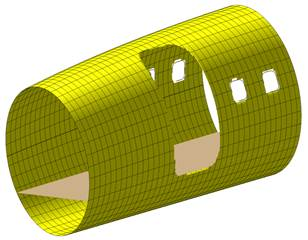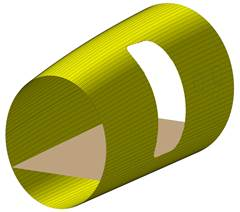Fig. 1: For a complex surface such an aircraft fuselage here, Needs high grid, therefore, the compilation is too long with modern computer (Intel Core i7, 2.4 GHz CPU, 16 GB RAM). If displays supplementary windows holes on fuselage, the software may bug when the RAM is full.

Question 1:

In matter of low cost computation, do you have better solution to create a fast surface with smooth hole from given a parametric surface equation S(x,y) and given any convex/concave-closed parametric curve C(t) that is projected on surface S(x,y)?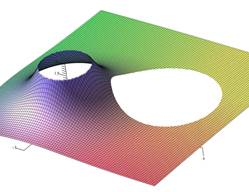Fig. 2: These surfaces are been created in Maple 2016 from given initial parametric surface equation and curve projected on the surface. But requires huge grid, huge RAM, and long time to get smooth hole when free CAD tools are fast and low RAM.

Question 2:

Because I noted that the Maple’s view option renderers smooth edges of any surface. My question is: Is it possible to use the internal structure of display/view used by Maple to apply at free boundaries? I think it will be possible to create many class of view, for examples: cylindrical sector, spherical sector views or more rather to be limited with conventional cubic view. And thus, I think it will be possible to create own smooth hole or multiple-hole on surface and get smooth pierced surface.

I know that almost of technicians/engineers will recommend me to use a CAD tool to create surface with hole. But the objective here is to keep purely a mathematical mind and work with exact equations (analytic equations).

On a free CAD tool, even the complex holes are created very fast only with low RAM. Why not on Maple?

Best.

Guy.

## Maple and Geometry Expressions software...

Hi, is anyone using the Geometry Expressions software together with Maple? I've found about this software on forum and installed a free demo version. It is using symbolic geometry, which I haven't been able to find this feature on other software, and can work very well with Maple, but unfortunatelly, my demo version doesn't work properly, and their oficial website http://saltire.com/ has lots of errors 404 page not found. I have requested support, but have had no answer so far. I was so happy finding this software, but now I am thinking maybe wasting my time. If you are using it, or maybe think it would be good to give it a go, please let me know if it is working for you. A particular feature which is not working for me is 'creating angles'. Thank you.

## How to write a the iteration code in Maple...

Dears I have the following statment in Matlab

r=8;             ZUM=U(1)-YU(1);            IT=0;

for r=2:10

ZU(r)=abs(U(r)-YU(r));

if ZU(r)> ZUM

ZUM=ZU(r);

else

ZUM=ZUM;

end

end

ZUM;

while IT < 20

IT=IT+1

if ZUM < (0.1)^r

IT=20;

else

for r=1:Nx

YU(r)=U(r);

end

YU;

I need to write this statment in Maple

## Correction on a Code...

Please I need Correction on this code particularly if I can make do without the declaration of vector in the third subroutine . The idea is to get maximum error. The code has 3 subroutine. The problem I think is in the third subroutine (Display of results).

Thank you in anticipation of positive response.

# First Declaration of the problem

restart:
Digits:=30:
interface(rtablesize=infinity):

f1:=proc(n)
y2[n]:
end proc:
f2:=proc(n)
-y1[n]+0.001*cos(t[n]):
end proc:
f3:=proc(n)
y4[n]:
end proc:
f4:=proc(n)
-y3[n]+0.001*sin(t[n]):
end proc:
F1:=proc(n)
f2(n):
end proc:
F2:=proc(n)
-(f1(n))-0.001*sin(t[n]):
end proc:
F3:=proc(n)
f4(n):
end proc:
F4:=proc(n)
-f3(n)+0.001*cos(t[n]):
end proc:

# Declaration of the Numerical methods

e1:=y1[n+2] = (7/23)*y1[n]+(16/23)*y1[n+1]+(12/23)*f1(n+2)*h+(16/23)*f1(n+1)*h-(2/23)*F1(n+2)*h^2+(2/23)*h*f1(n)+((24/3703)*y1[n]-(24/3703)*y1[n+1]+(48/18515)*f1(n+2)*h+(8/55545)*f1(n+1)*h-(116/55545)*F1(n+2)*h^2+(208/55545)*h*f1(n))*u^2+((901/2980915)*y1[n]-(901/2980915)*y1[n+1]+(7109/89427450)*f1(n+2)*h+(923/14904575)*f1(n+1)*h-(6241/89427450)*F1(n+2)*h^2+(14383/89427450)*h*f1(n))*u^4+((1979723/158376013950)*y1[n]-(1979723/158376013950)*y1[n+1]+(6364571/2375640209250)*f1(n+2)*h+(728327/215967291750)*f1(n+1)*h-(11785633/4751280418500)*F1(n+2)*h^2+(5106559/791880069750)*h*f1(n))*u^6+((6488435581/13259239887894000)*y1[n]-(6488435581/13259239887894000)*y1[n+1]+(8693517709/91794737685420000)*f1(n+2)*h+(260601208141/1789997384865690000)*f1(n+1)*h-(323357994149/3579994769731380000)*F1(n+2)*h^2+(891627999937/3579994769731380000)*h*f1(n))*u^8+((25090513463/1343541160668420000)*y1[n]-(25090513463/1343541160668420000)*y1[n+1]+(190450718149/55421072877572325000)*f1(n+2)*h+(47563947061/8210529315195900000)*f1(n+1)*h-(1475729910283/443368583020578600000)*F1(n+2)*h^2+(261738159769/27710536438786162500)*h*f1(n))*u^10+((244426606265778733/347060946154014557665200000)*y1[n]-(244426606265778733/347060946154014557665200000)*y1[n+1]+(1316372988977975777/10411828384620436729956000000)*f1(n+2)*h+(105391490263288387/473264926573656214998000000)*f1(n+1)*h-(1284959669761615073/10411828384620436729956000000)*F1(n+2)*h^2+(72506125749079249/204153497737655622156000000)*h*f1(n))*u^12:

e2:=h^2*F1(n+1) = (60/23)*y1[n]-(60/23)*y1[n+1]+(25/46)*f1(n+2)*h+(32/23)*f1(n+1)*h-(4/23)*F1(n+2)*h^2+(31/46)*h*f1(n)+((209/3703)*y1[n]-(209/3703)*y1[n+1]+(1313/222180)*f1(n+2)*h+(1304/55545)*f1(n+1)*h-(131/18515)*F1(n+2)*h^2+(6011/222180)*h*f1(n))*u^2+((77491/35770980)*y1[n]-(77491/35770980)*y1[n+1]+(574843/2146258800)*f1(n+2)*h+(113536/134141175)*f1(n+1)*h-(53461/178854900)*F1(n+2)*h^2+(2258041/2146258800)*h*f1(n))*u^4+((151508243/1900512167400)*y1[n]-(151508243/1900512167400)*y1[n+1]+(1290306599/114030730044000)*f1(n+2)*h+(18919693/647901875250)*f1(n+1)*h-(113769323/9502560837000)*F1(n+2)*h^2+(4470322013/114030730044000)*h*f1(n))*u^6+((42120775181/14464625332248000)*y1[n]-(42120775181/14464625332248000)*y1[n+1]+(332746636891/734357901483360000)*f1(n+2)*h+(302396120633/298332897477615000)*f1(n+1)*h-(369019384141/795554393273640000)*F1(n+2)*h^2+(13797329479621/9546652719283680000)*h*f1(n))*u^8+((18953368786273/177347433208231440000)*y1[n]-(18953368786273/177347433208231440000)*y1[n+1]+(2430202319484337/138330997902420523200000)*f1(n+2)*h+(310803544671199/8645687368901282700000)*f1(n+1)*h-(203453960588449/11527583158535043600000)*F1(n+2)*h^2+(7380568619069419/138330997902420523200000)*h*f1(n))*u^10+((16436168060905785763/4164731353848174691982400000)*y1[n]-(16436168060905785763/4164731353848174691982400000)*y1[n+1]+(167160345356705269819/249883881230890481518944000000)*f1(n+2)*h+(461636091223370027/354948694930242161248500000)*f1(n+1)*h-(13852288092290788813/20823656769240873459912000000)*F1(n+2)*h^2+(29059878239787610409/14699051837111204795232000000)*h*f1(n))*u^12:

e3:=y2[n+2] = (7/23)*y2[n]+(16/23)*y2[n+1]+(12/23)*f2(n+2)*h+(16/23)*f2(n+1)*h-(2/23)*F2(n+2)*h^2+(2/23)*h*f2(n)+((24/3703)*y2[n]-(24/3703)*y2[n+1]+(48/18515)*f2(n+2)*h+(8/55545)*f2(n+1)*h-(116/55545)*F2(n+2)*h^2+(208/55545)*h*f2(n))*u^2+((901/2980915)*y2[n]-(901/2980915)*y2[n+1]+(7109/89427450)*f2(n+2)*h+(923/14904575)*f2(n+1)*h-(6241/89427450)*F2(n+2)*h^2+(14383/89427450)*h*f2(n))*u^4+((1979723/158376013950)*y2[n]-(1979723/158376013950)*y2[n+1]+(6364571/2375640209250)*f2(n+2)*h+(728327/215967291750)*f2(n+1)*h-(11785633/4751280418500)*F2(n+2)*h^2+(5106559/791880069750)*h*f2(n))*u^6+((6488435581/13259239887894000)*y2[n]-(6488435581/13259239887894000)*y2[n+1]+(8693517709/91794737685420000)*f2(n+2)*h+(260601208141/1789997384865690000)*f2(n+1)*h-(323357994149/3579994769731380000)*F2(n+2)*h^2+(891627999937/3579994769731380000)*h*f2(n))*u^8+((25090513463/1343541160668420000)*y2[n]-(25090513463/1343541160668420000)*y2[n+1]+(190450718149/55421072877572325000)*f2(n+2)*h+(47563947061/8210529315195900000)*f2(n+1)*h-(1475729910283/443368583020578600000)*F2(n+2)*h^2+(261738159769/27710536438786162500)*h*f2(n))*u^10+((244426606265778733/347060946154014557665200000)*y2[n]-(244426606265778733/347060946154014557665200000)*y2[n+1]+(1316372988977975777/10411828384620436729956000000)*f2(n+2)*h+(105391490263288387/473264926573656214998000000)*f2(n+1)*h-(1284959669761615073/10411828384620436729956000000)*F2(n+2)*h^2+(72506125749079249/204153497737655622156000000)*h*f2(n))*u^12:

e4:=h^2*F2(n+1) = (60/23)*y2[n]-(60/23)*y2[n+1]+(25/46)*f2(n+2)*h+(32/23)*f2(n+1)*h-(4/23)*F2(n+2)*h^2+(31/46)*h*f2(n)+((209/3703)*y2[n]-(209/3703)*y2[n+1]+(1313/222180)*f2(n+2)*h+(1304/55545)*f2(n+1)*h-(131/18515)*F2(n+2)*h^2+(6011/222180)*h*f2(n))*u^2+((77491/35770980)*y2[n]-(77491/35770980)*y2[n+1]+(574843/2146258800)*f2(n+2)*h+(113536/134141175)*f2(n+1)*h-(53461/178854900)*F2(n+2)*h^2+(2258041/2146258800)*h*f2(n))*u^4+((151508243/1900512167400)*y2[n]-(151508243/1900512167400)*y2[n+1]+(1290306599/114030730044000)*f2(n+2)*h+(18919693/647901875250)*f2(n+1)*h-(113769323/9502560837000)*F2(n+2)*h^2+(4470322013/114030730044000)*h*f2(n))*u^6+((42120775181/14464625332248000)*y2[n]-(42120775181/14464625332248000)*y2[n+1]+(332746636891/734357901483360000)*f2(n+2)*h+(302396120633/298332897477615000)*f2(n+1)*h-(369019384141/795554393273640000)*F2(n+2)*h^2+(13797329479621/9546652719283680000)*h*f2(n))*u^8+((18953368786273/177347433208231440000)*y2[n]-(18953368786273/177347433208231440000)*y2[n+1]+(2430202319484337/138330997902420523200000)*f2(n+2)*h+(310803544671199/8645687368901282700000)*f2(n+1)*h-(203453960588449/11527583158535043600000)*F2(n+2)*h^2+(7380568619069419/138330997902420523200000)*h*f2(n))*u^10+((16436168060905785763/4164731353848174691982400000)*y2[n]-(16436168060905785763/4164731353848174691982400000)*y2[n+1]+(167160345356705269819/249883881230890481518944000000)*f2(n+2)*h+(461636091223370027/354948694930242161248500000)*f2(n+1)*h-(13852288092290788813/20823656769240873459912000000)*F2(n+2)*h^2+(29059878239787610409/14699051837111204795232000000)*h*f2(n))*u^12:

e5:=y3[n+2] = (7/23)*y3[n]+(16/23)*y3[n+1]+(12/23)*f3(n+2)*h+(16/23)*f3(n+1)*h-(2/23)*F3(n+2)*h^2+(2/23)*h*f3(n)+((24/3703)*y3[n]-(24/3703)*y3[n+1]+(48/18515)*f3(n+2)*h+(8/55545)*f3(n+1)*h-(116/55545)*F3(n+2)*h^2+(208/55545)*h*f3(n))*u^2+((901/2980915)*y3[n]-(901/2980915)*y3[n+1]+(7109/89427450)*f3(n+2)*h+(923/14904575)*f3(n+1)*h-(6241/89427450)*F3(n+2)*h^2+(14383/89427450)*h*f3(n))*u^4+((1979723/158376013950)*y3[n]-(1979723/158376013950)*y3[n+1]+(6364571/2375640209250)*f3(n+2)*h+(728327/215967291750)*f3(n+1)*h-(11785633/4751280418500)*F3(n+2)*h^2+(5106559/791880069750)*h*f3(n))*u^6+((6488435581/13259239887894000)*y3[n]-(6488435581/13259239887894000)*y3[n+1]+(8693517709/91794737685420000)*f3(n+2)*h+(260601208141/1789997384865690000)*f3(n+1)*h-(323357994149/3579994769731380000)*F3(n+2)*h^2+(891627999937/3579994769731380000)*h*f3(n))*u^8+((25090513463/1343541160668420000)*y3[n]-(25090513463/1343541160668420000)*y3[n+1]+(190450718149/55421072877572325000)*f3(n+2)*h+(47563947061/8210529315195900000)*f3(n+1)*h-(1475729910283/443368583020578600000)*F3(n+2)*h^2+(261738159769/27710536438786162500)*h*f3(n))*u^10+((244426606265778733/347060946154014557665200000)*y3[n]-(244426606265778733/347060946154014557665200000)*y3[n+1]+(1316372988977975777/10411828384620436729956000000)*f3(n+2)*h+(105391490263288387/473264926573656214998000000)*f3(n+1)*h-(1284959669761615073/10411828384620436729956000000)*F3(n+2)*h^2+(72506125749079249/204153497737655622156000000)*h*f3(n))*u^12:
e6:=h^2*F3(n+1) = (60/23)*y3[n]-(60/23)*y3[n+1]+(25/46)*f3(n+2)*h+(32/23)*f3(n+1)*h-(4/23)*F3(n+2)*h^2+(31/46)*h*f3(n)+((209/3703)*y3[n]-(209/3703)*y3[n+1]+(1313/222180)*f3(n+2)*h+(1304/55545)*f3(n+1)*h-(131/18515)*F3(n+2)*h^2+(6011/222180)*h*f3(n))*u^2+((77491/35770980)*y3[n]-(77491/35770980)*y3[n+1]+(574843/2146258800)*f3(n+2)*h+(113536/134141175)*f3(n+1)*h-(53461/178854900)*F3(n+2)*h^2+(2258041/2146258800)*h*f3(n))*u^4+((151508243/1900512167400)*y3[n]-(151508243/1900512167400)*y3[n+1]+(1290306599/114030730044000)*f3(n+2)*h+(18919693/647901875250)*f3(n+1)*h-(113769323/9502560837000)*F3(n+2)*h^2+(4470322013/114030730044000)*h*f3(n))*u^6+((42120775181/14464625332248000)*y3[n]-(42120775181/14464625332248000)*y3[n+1]+(332746636891/734357901483360000)*f3(n+2)*h+(302396120633/298332897477615000)*f3(n+1)*h-(369019384141/795554393273640000)*F3(n+2)*h^2+(13797329479621/9546652719283680000)*h*f3(n))*u^8+((18953368786273/177347433208231440000)*y3[n]-(18953368786273/177347433208231440000)*y3[n+1]+(2430202319484337/138330997902420523200000)*f3(n+2)*h+(310803544671199/8645687368901282700000)*f3(n+1)*h-(203453960588449/11527583158535043600000)*F3(n+2)*h^2+(7380568619069419/138330997902420523200000)*h*f3(n))*u^10+((16436168060905785763/4164731353848174691982400000)*y3[n]-(16436168060905785763/4164731353848174691982400000)*y3[n+1]+(167160345356705269819/249883881230890481518944000000)*f3(n+2)*h+(461636091223370027/354948694930242161248500000)*f3(n+1)*h-(13852288092290788813/20823656769240873459912000000)*F3(n+2)*h^2+(29059878239787610409/14699051837111204795232000000)*h*f3(n))*u^12:

e7:=y4[n+2] = (7/23)*y4[n]+(16/23)*y4[n+1]+(12/23)*f4(n+2)*h+(16/23)*f4(n+1)*h-(2/23)*F4(n+2)*h^2+(2/23)*h*f4(n)+((24/3703)*y4[n]-(24/3703)*y4[n+1]+(48/18515)*f4(n+2)*h+(8/55545)*f4(n+1)*h-(116/55545)*F4(n+2)*h^2+(208/55545)*h*f4(n))*u^2+((901/2980915)*y4[n]-(901/2980915)*y4[n+1]+(7109/89427450)*f4(n+2)*h+(923/14904575)*f4(n+1)*h-(6241/89427450)*F4(n+2)*h^2+(14383/89427450)*h*f4(n))*u^4+((1979723/158376013950)*y4[n]-(1979723/158376013950)*y4[n+1]+(6364571/2375640209250)*f4(n+2)*h+(728327/215967291750)*f4(n+1)*h-(11785633/4751280418500)*F4(n+2)*h^2+(5106559/791880069750)*h*f4(n))*u^6+((6488435581/13259239887894000)*y4[n]-(6488435581/13259239887894000)*y4[n+1]+(8693517709/91794737685420000)*f4(n+2)*h+(260601208141/1789997384865690000)*f4(n+1)*h-(323357994149/3579994769731380000)*F4(n+2)*h^2+(891627999937/3579994769731380000)*h*f4(n))*u^8+((25090513463/1343541160668420000)*y4[n]-(25090513463/1343541160668420000)*y4[n+1]+(190450718149/55421072877572325000)*f4(n+2)*h+(47563947061/8210529315195900000)*f4(n+1)*h-(1475729910283/443368583020578600000)*F4(n+2)*h^2+(261738159769/27710536438786162500)*h*f4(n))*u^10+((244426606265778733/347060946154014557665200000)*y4[n]-(244426606265778733/347060946154014557665200000)*y4[n+1]+(1316372988977975777/10411828384620436729956000000)*f4(n+2)*h+(105391490263288387/473264926573656214998000000)*f4(n+1)*h-(1284959669761615073/10411828384620436729956000000)*F4(n+2)*h^2+(72506125749079249/204153497737655622156000000)*h*f4(n))*u^12:

e8:=h^2*F4(n+1) = (60/23)*y4[n]-(60/23)*y4[n+1]+(25/46)*f4(n+2)*h+(32/23)*f4(n+1)*h-(4/23)*F4(n+2)*h^2+(31/46)*h*f4(n)+((209/3703)*y4[n]-(209/3703)*y4[n+1]+(1313/222180)*f4(n+2)*h+(1304/55545)*f4(n+1)*h-(131/18515)*F4(n+2)*h^2+(6011/222180)*h*f4(n))*u^2+((77491/35770980)*y4[n]-(77491/35770980)*y4[n+1]+(574843/2146258800)*f4(n+2)*h+(113536/134141175)*f4(n+1)*h-(53461/178854900)*F4(n+2)*h^2+(2258041/2146258800)*h*f4(n))*u^4+((151508243/1900512167400)*y4[n]-(151508243/1900512167400)*y4[n+1]+(1290306599/114030730044000)*f4(n+2)*h+(18919693/647901875250)*f4(n+1)*h-(113769323/9502560837000)*F4(n+2)*h^2+(4470322013/114030730044000)*h*f4(n))*u^6+((42120775181/14464625332248000)*y4[n]-(42120775181/14464625332248000)*y4[n+1]+(332746636891/734357901483360000)*f4(n+2)*h+(302396120633/298332897477615000)*f4(n+1)*h-(369019384141/795554393273640000)*F4(n+2)*h^2+(13797329479621/9546652719283680000)*h*f4(n))*u^8+((18953368786273/177347433208231440000)*y4[n]-(18953368786273/177347433208231440000)*y4[n+1]+(2430202319484337/138330997902420523200000)*f4(n+2)*h+(310803544671199/8645687368901282700000)*f4(n+1)*h-(203453960588449/11527583158535043600000)*F4(n+2)*h^2+(7380568619069419/138330997902420523200000)*h*f4(n))*u^10+((16436168060905785763/4164731353848174691982400000)*y4[n]-(16436168060905785763/4164731353848174691982400000)*y4[n+1]+(167160345356705269819/249883881230890481518944000000)*f4(n+2)*h+(461636091223370027/354948694930242161248500000)*f4(n+1)*h-(13852288092290788813/20823656769240873459912000000)*F4(n+2)*h^2+(29059878239787610409/14699051837111204795232000000)*h*f4(n))*u^12:

# Display of the solutions

h:=evalf(Pi/6):

omega:=1.0:
u:=omega*h:
N:=solve(h*p = 12*Pi/6, p):
n:=0:

exy1:= [seq](eval(cos(i)+0.0005*i*sin(i)), i=h..N,h):
exy2:= [seq](eval(-0.9995*sin(i)+0.0005), i=h..N,h):
exy3:= [seq](eval(sin(i)-0.0005*i*cos(i)), i=h..N,h):
exy4:= [seq](eval(0.9995*sin(i)+0.0005*i*sin(i)), i=h..N,h):

iny1:=1:
iny2:=0:
iny3:=0:
iny4:=0.9995:

err1 := Vector(N):
err2 := Vector(N):
c:=1:
inx:=0:
vars := y1[n+1],y1[n+2],y2[n+1],y2[n+2],y3[n+1],y3[n+2],y4[n+1],y4[n+2]:
for j from 0 to 2 do
x[j]:=inx+j*h:
end do:
printf("%4s%9s%9s%9s%9s%9s%9s%10s%10s%9s%9s%9s%10s\n",
"h","numy1","numy2","numy3","numy4",
"exy1","exy2","exy3","exy4",
"erry1","erry2","erry3","erry4");

st := time():
for k from 1 to N/2 do
param1:=y1[n]=iny1,y2[n]=iny2,y3[n]=iny3,y4[n]=iny4:
param2:=t[n]=x,t[n+1]=x,t[n+2]=x:

res:=eval(<vars>, fsolve(eval({e||(1..8)},[param1,param2]),{vars})):

for i from 1 to 2 do
printf("%5.2f%9.3f%9.3f%9.3f%9.3f %8.5f%10.5f%10.5f%10.5f %8.2g%9.3g%9.3g%8.3g\n",
h*c,res[i],res[i+2],res[i+4],res[i+6],
exy1[c],exy2[c],exy3[c],exy4[c],
abs(res[i]-exy1[c]),abs(res[i+2]-exy2[c]),abs(res[i+4]-exy3[c]),abs(res[i+6]-exy4[c])):

err1[c] := abs(evalf(res[i]-exy1)):
err2[c] := abs(evalf(res[i+4]-exy3)):
c:=c+1:
end do:
iny1:=res:
iny2:=res:
iny3:=res:
iny4:=res:
inx:=x:
for j from 0 to 2 do
x[j]:=inx+j*h:
end do:
end do:
v:=time() - st;
printf("Maximum error is %.13g", max(err1));
printf("Maximum error is %.13g", max(err2));

## Error in numerical solution of nonlinear ode...

i tried to solve a nonlinear ode with numerical method but maple can't solve it and this error occur:

Error, (in dsolve/numeric/bvp) initial Newton iteration is not converging

my maple codes are attached below:

numeriacal_sol.mw

can any help me?

## how i can solve following equation?...

hi--how i can solve following equation?

thanks

Eq.mw

Maple Worksheet - Error

Failed to load the worksheet /maplenet/convert/Eq.mw .

## How do I speed up the Evaluation of Elliptical fun...

Question:- can the procedure given below called "epi" be speeded up by compiling/ using evhf.If so how? My paple code is at the bottom.

First some background information.

Recently I ran into a difference in usage of a couple of elliptical functions between Maple and Mathematica.  This first case concerned EllipticalPi. The author of the blog kindly wrote  a Maple procedure to produce the same results as Mathematica’s  usage of ElllipticalPi.

I tested the basic integral that produces the EllipticPi    Ell := int(1/(1-nu*JacobiSN(t, k)^2), t)  answer      Ell := EllipticPi(JacobiSN(t, k), nu, k). They do not produce the same outcome. Plots are in the document .  They agree in one quarter only.

I then ran into a difference in usage of  EllipticF. This time I was able to get to same outcome myself using Maple’s help.

“It is worth noting the difference between the Legendre normal form of the Incomplete Elliptic integral of the first kind (see A&S 17.2.7), in Maple represented by EllipticF(z,k) but for the splitting of the square root in the denominator of the integrand (see definition lines above), and the normal trigonometric form of this elliptic integral (see A&S 17.2.6), in Maple represented by the InverseJacobiAM function
InverseJacobiAM(phi,k);

That worked fine.

There is no mention in the help for usage implementation of EllipticPi as opposed to different usages as there is with EllipticF. I do not know if there is a way in Maple of achieving the same enactment as Mathematica in this case, without the Procedure I  was  given.

Elliptic Pi in Mathematica and Maple

We use EllipticPi when we write exact solutions of rotation of a free asymmetric top. While solving Euler’s equations for angular velocity or angular momentum in the body frame we need Jacobi elliptic functions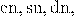solving the differential equation for the attitude matrix involves EllipticPi function. As I have explained it in Taming the T-handle continued we need the integral

(1)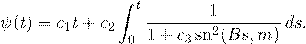In Mathematica this is easily implemented as

(2)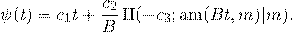However, as pointed out by Rowan in a comment to Taming the T-handle continued , the same formula does not work with Maple.

While the documentations of both Mathematica and Maple contain links to Abramowitz and Stegun Handbook of Mathematical Functions, they use different definitions. Here is what concerns us, from p. 590 of the 10th printing: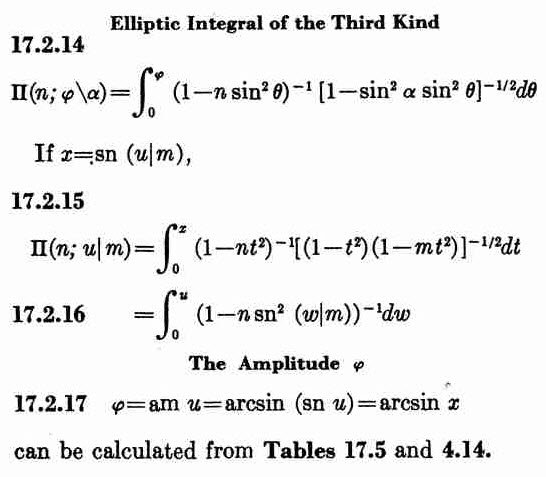What we need is 17.2.16, while Maple is using 17.2.14. To convert we need to set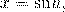but such a conversion is possible only in the domain where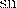can be inverted. We can do it easily for sufficiently small values of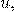but not necessarily for values that contain several quarter-periods.

The following Maple procedure does the job:

epi := proc (t::float, nu::float, k::float) local t2, n, dt, ep0, res; ep0 := EllipticPi(nu, k); t2 := EllipticK(k); n := floor(t/t2); dt := t-t2*n; if type(n, even) then res := Re(n*ep0+EllipticPi(JacobiSN(dt, k), nu, k)) else res := Re((1+n)*ep0-EllipticPi(JacobiSN(t2-dt, k), nu, k)) end if end proc

HAs an example here is the Maple plot for nu=-3, k=0.9:
plot(('epi')(t, -3.0, .9), t = -20 .. 20)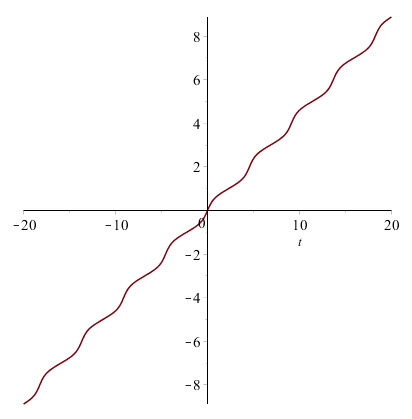And here is the corresponding Mathematica plot: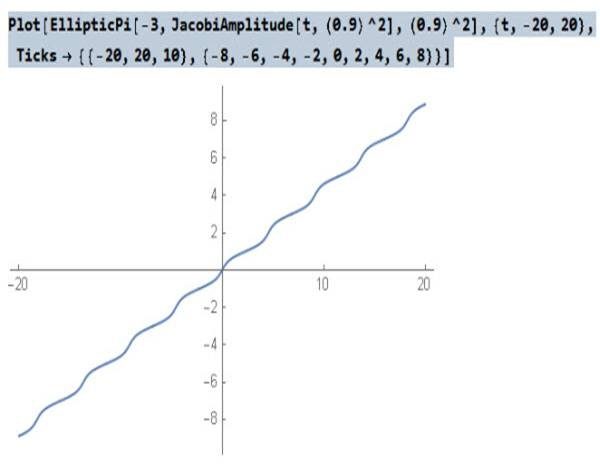The function epi(t,nu, k) defined above for Maple gives now the same result as EllipticPi(nu,JacobiAM(t,k^2),k^2) in Mathematica.

restart;
epi := proc (t, nu, k) local t2, n, dt, ep0, res; ep0 := EllipticPi(nu, k); t2 := EllipticK(k); n := floor(t/t2); dt := t-t2*n; if type(n, even) then res := Re(n*ep0+EllipticPi(JacobiSN(dt, k), nu, k)) else res := Re((1+n)*ep0-EllipticPi(JacobiSN(t2-dt, k), nu, k)) end if end proc;

Ell := int(1/(1-nu*JacobiSN(t, k)^2), t);
Ell := EllipticPi(JacobiSN(t, k), nu, k)
k := .9;
k := 0.9
nu := -3;
nu := -3
plot([epi(t, nu, k), Ell], t = -8 .. 20);

## Maple 2017: What to expect?...

Is there anyone who has seen maple 2017 provide some details about what new features are being introduced. Is there a platform where we can suggest what features we would like to be added or enhanced?

## Multi-threaded parallel programming a nested for l...

I have a nested for loop that iterates through a range of values for x and y coordinates to create a 3d surface for illustration of my research. after the x loop there is a y loop, and inside of that y loop is a series of commands to find some eigenvalues of a matrix (which become the z coordinates) and sort them into already open files. This isn't bad when the precision i require is more than .02, but some of my matrices require up to 0.005 or less. The latter precision costs hours of computation time on just one processor. However my laptop has an i7, so I want to see if i can get the for loop to send its next iteration to the next processor in line while it has the previous ones still calculating. Have any tips?